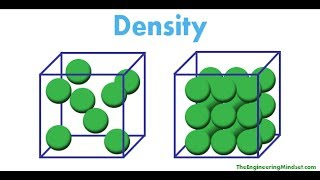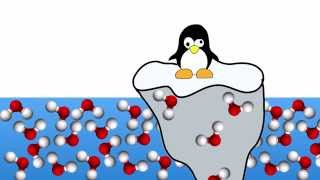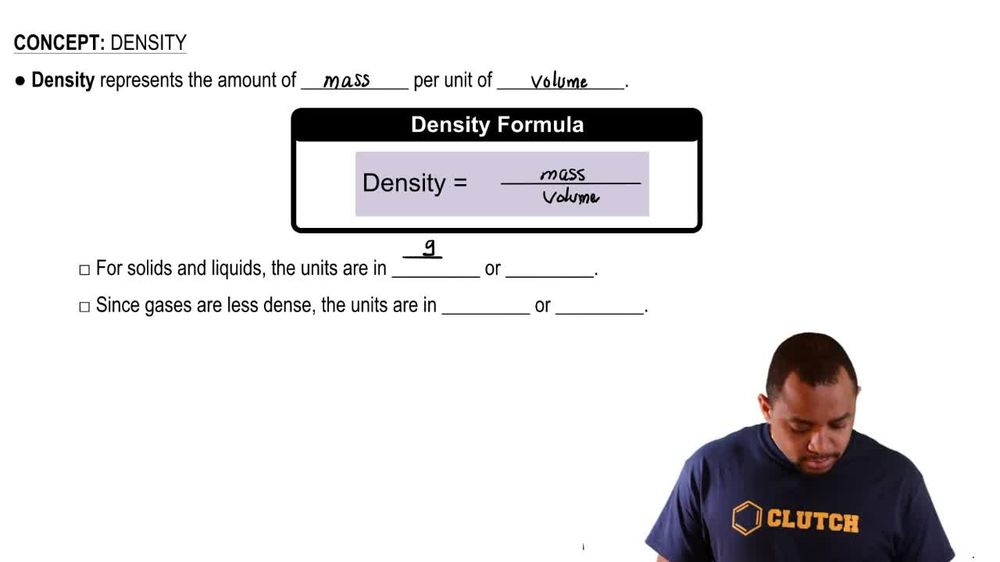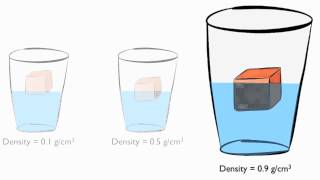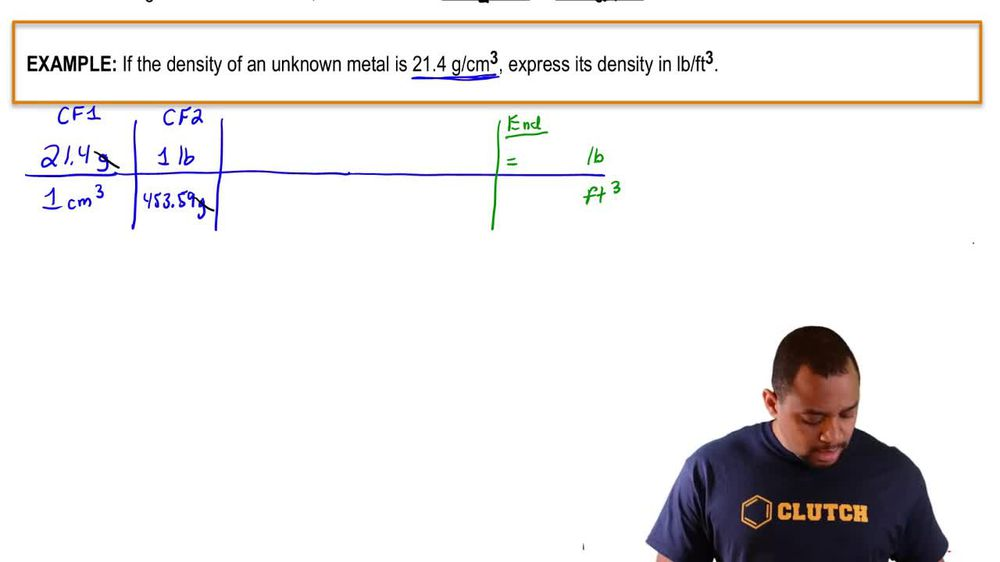Start typing, then use the up and down arrows to select an option from the list.
1. 1. Intro to General Chemistry2. Density
Problem

# (b) Carbon dioxide 1CO22 is a gas at room temperature and pressure. However, carbon dioxide can be put under pressure to become a “supercritical fluid” that is a much safer dry-cleaning agent than tetrachloroethylene. At a certain pressure, the density of supercritical CO2 is 0.469 g>cm3. What is the mass of a 25.0-mL sample of supercritical CO2 at this pressure?

Relevant Solution3m
Play a video:
Hey everyone. So here it says a lake with a surface area of 500 miles squared and an average depth of 15 ft is polluted with 150.3 micrograms of mercury per millimeter of mercury, calculate the total mass in kilograms of mercury in the lake. Alright, so here, what we need to do first is we need to basically get the units to match up. Our density is in micrograms per mil leader. And here we have units of length. Remember that centimeters cubed, one centimeters cubed is equal to one millimeter. So that's a clear giveaway that I need to convert miles squared and feet both into centimeters and then multiply them together to get centimeters cubed. So we're gonna start out with our 500 miles squared. And the conversion factor is that one kilometer is equal 2.621371 miles here I squared so that the mile square cancel out. And then we're gonna stay here, one km is 10 to the three m. And here we also have to square this. And then we'd say finally that one cm is equal to 10 to the -2 m. This comes out to 1.294 9, 9 times 10 to the 13 cm squared. Next I would take my 15 ft and I'd convert that also into cm. So remember one ft is 12", 1" is 2.54 cm. So that gives me 457.2 cm. Now when we multiply these centimeters squared by this centimeter, multiplying them together gives me centimeters cubed that comes out to be 5.9207 times 10 to the 15 centimeters cubed. So again it comes from multiplying this number with this number Now that I have centimeters cubed. Remember centimeters cubed is the same thing as Ml So we're gonna have . micrograms of mercury per one million. And then one microgram is equal to 10 to the -6 g. And then finally one kg is 10 to the six g or to the three g. Sorry, 10 to the three g. So that comes out to be 1.77, 6 times 10 to the six kg. Now here we want it in terms of sick fix. Let's see. 500 has won six fig. But that's a lot of rounding 15 ft. And then .3 also has one sig fig. So ideally we want to do it in terms of one sig fig. And that's gonna give us the best answer. So here, if we were doing in terms of six figs, that comes out to 22 times 10 to the six kg. Okay, so that would be the answer with the correct number of significant figures